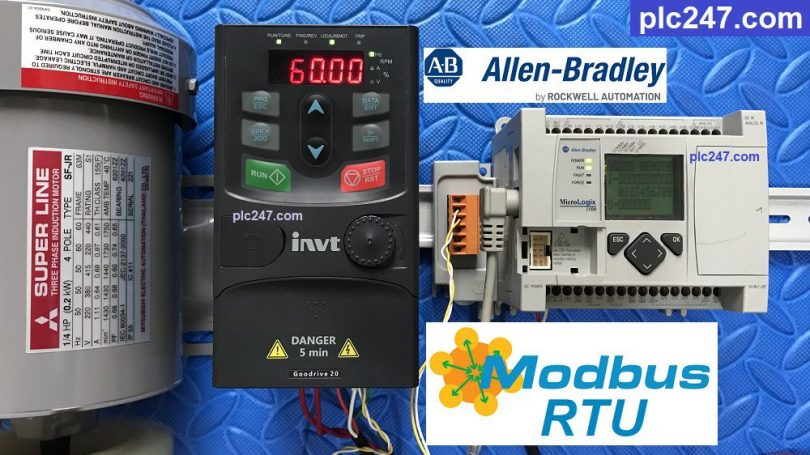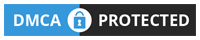# Micrologix 1100 “Modbus RTU” INVT GD20 VFDWritten by

Hello, today plc247.com continues to guide you to connect Allen Bradley MicroLogix Series PLC to INVT GD20 VFD using “Modbus RTU” Communication Standard.

The PLC model I use is MicroLogix 1100 combined with Cable RS232/RS485 1763-NC01 (if you don’t have one, you can plug it directly into the port of the PLC)

#### INVT Goodrive20 Modbus RTU Settings

In addition to the basic parameters of the inverter installed according to the motor (frequency, current, voltage), we need to set the communication parameters as follows:

• P00.01 = 2 (Frequency Reference via RS485)
• P00.06 = 8 (Motor Control via RS485)
• P00.09 = 0 (A Frequency Selection)
• P14.00 = 1 (Slave Address: 1)
• P14.01 = 3 (9600bps)
• P14.02 = 1 (Modbus RTU 8/E/1)
• P14.03 = 5
• P14.04 = 0.0
##### Register for Control

+ Motor Control Address = 2000 (hex) + 1
= 8192 (dec) + 1 = 8193

• Set Word 8193 = 1 >>> Forward Run
= 2 >>> Reverse Run
= 5 >>> Stop

+ Frequency Reference Address = 2001 (hex) + 1
= 8193 + 1 = 8194

##### Register for Monitoring
• Output Frequency Address = 3000 (hex) + 1 = 12288 (dec) + 1 = 12289
• Setting Frequency Address = 12290
• DC-BUS Voltage Address = 12291
• Output Volatge Address = 12292
• Output Current Address = 12293

### PLC Programming

+ Channel Configuration

+ Generate 0.01s Pulse and Read/Write Distribution

+ Motor Control Command

+ Frequency Reference Command

+ Data Monitoring Command

### Video Tutorial

======

Required Documentation